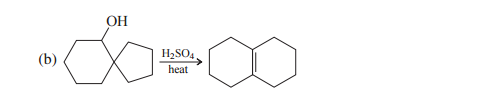# Problem: Write a sequence of steps depicting the mechanisms of each of the following reactions. Use curved arrows to show electron flow.

###### Problem Details

Write a sequence of steps depicting the mechanisms of each of the following reactions. Use curved arrows to show electron flow.What scientific concept do you need to know in order to solve this problem?

Our tutors have indicated that to solve this problem you will need to apply the Dehydration Reaction concept. You can view video lessons to learn Dehydration Reaction. Or if you need more Dehydration Reaction practice, you can also practice Dehydration Reaction practice problems.

What is the difficulty of this problem?

Our tutors rated the difficulty ofWrite a sequence of steps depicting the mechanisms of each o...as high difficulty.

How long does this problem take to solve?

Our expert Organic tutor, Chris took 4 minutes and 38 seconds to solve this problem. You can follow their steps in the video explanation above.

What textbook is this problem found in?

Our data indicates that this problem or a close variation was asked in Organic Chemistry - Carey 8th Edition. You can also practice Organic Chemistry - Carey 8th Edition practice problems.